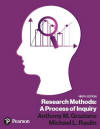﻿ Logic of Inferential StatisticsGraziano & Raulin
Research Methods (9th edition)

## Logic of Inferential Statistics

Being able to describe your data with descriptive statistics is quite useful, but the real heart of statistics is inferential statistics, which allow researchers to make decisions about the meaning of their data. Chapter 5 introduced you to the basic concepts of inferential statistics, as well as its logic and terminology. This section will go well beyond that brief description.

The first topic in this section is the concept of probability. Probability provides the underpinning for all inferential statistics.

The second topic is sampling and sampling distributions.

These are not easy topics for most students, but they are not impossible either. If you take your time and make sure you understand them, you will find that the third topic of this section (inferential statistics) is simply a natural extension of these discussions of probability and sampling distributions.

Click on the Next Page button below to begin this section, or click on any of the individual sections in the Table of Contents below to go right to that section.

 Probability Sampling Distributions Inferential Statistics